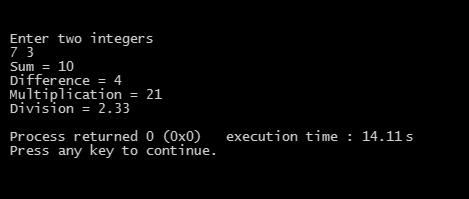## C Programming Examples Tutorial Index

C program to perform basic arithmetic operations of two numbers. Numbers are assumed to be integers and will be entered by the user.

``````#include <stdio.h>
int main()
{
int first, second, add, subtract, multiply;
float divide;

printf("Enter two integers\n");
scanf("%d%d", &first, &second);
subtract = first - second;
multiply = first * second;
divide     = first / (float)second;   //typecasting